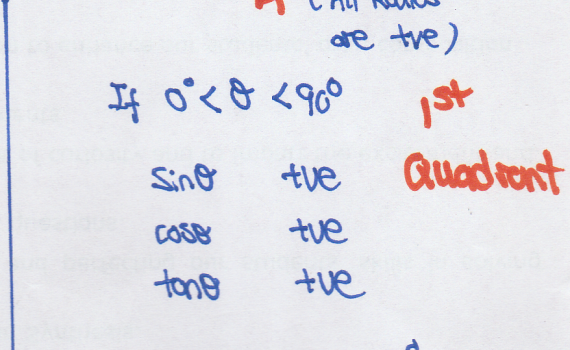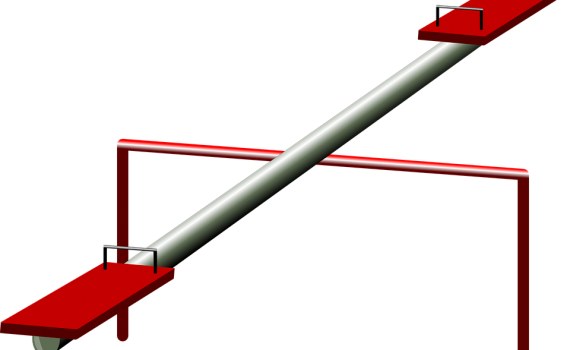# Resources

## Sine Cosine AnglesTrigonometric Ratios: ASTC To remember the different signs in each quadrant, use ASTC. All Science Teachers are Crazy. Printer Friendly  Trigo Ratios ASTC

## Definite IntegrationInverse Proportion: Effects on  y  when  x  increases Given that  y  is inversely proportional to the cube root of  x .  Find the percentage decrease in  y  when  x  is increased by 300%. Solution